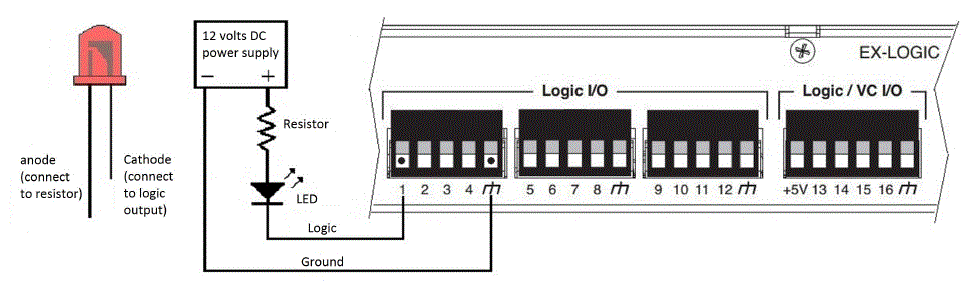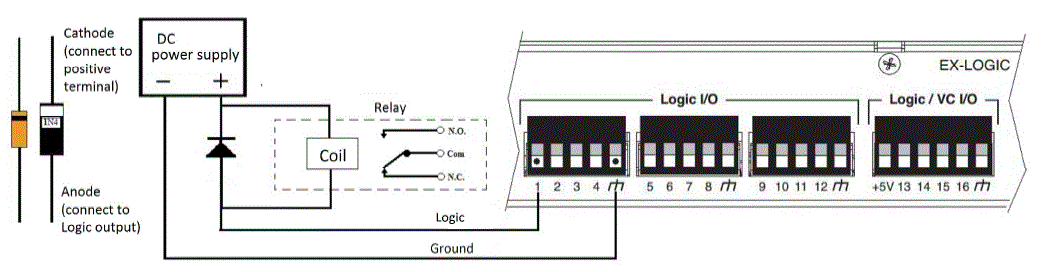# Wiring LED's and relays to the EX-LOGIC

Besides providing the capability of using external devices, such as switches and potentiometer to control Tesira, there’s the possibility of having the audio system control external devices such as LEDs, relays, or drive logic inputs of other devices. LEDs and Relays can be connected to Tesira thru an EX-Logic or via GPIO pins on other hardware.

This note covers the details on wiring LEDs and Relays to the EX-Logic

## Wiring an LED

An LED can be connected to an EX-LOGIC such that it is being powered directly by the EX-LOGIC, or powered from an external power supply.

### Using EX-LOGIC as a power source

When configured as a logic output, each of the 16 GPIO pins can be configured as a current source to drive an LED directly (5V / 10mA maximum per output). To wire an LED in this mode, connect the LED’s anode (long lead) to the EX-Logic output and the cathode (short lead) to the ground terminal on the EX-logic as shown in the diagram below:When using EX-LOGIC as a power source, the corresponding Logic Output blocks in your Tesira configuration must be created with the "Enable Powered Outputs" option selected.

Additionally, four of the connections (13-16) are configurable to operate as variable voltage control connections. These connections can provide a variable output from ground up to +5V. They can also accept a variable input voltage (such as a potentiometer for volume control) up to 5V, which can be sourced from the +5V port next to port 13.

## Using an external power source

GPIO pins 1-12 can be configured as simple contact closure outputs capable of sinking up to 300mA at 40V. In this mode, an external power supply is required (e.g. to drive a high brightness LED or multiple LED’s). GPIO pins 13-16 are not capable of sinking voltages greater than 4V and must use an external relay; GPIO pin behavior on Server, Server-IO, Forte are the same as EX-Logic pins 13-16. (see below).

Please note: When LED’s are wired to the EX-Logic using this method, the “Enable Powered Outputs” should remain unchecked in the Logic Output Initialization window in Tesira to avoid damaging the EX-Logic.

To wire an LED to an EX-logic using an external power supply, make the following connections as shown in the diagram below:

1. Connect the negative terminal of the power supply to the ground terminal on the EX-Logic.
2. Connect the positive terminal of the power supply to the resistor. Resistor value can be calculated using the following formula:

R = V/I

where:

R = value of resistor in kOhms
V = supply voltage – the LED turn on voltage
I  = required current in mA
1. Connect the resistor to the LED’s Anode (long lead).
2. Connect the LED’s Cathode (short lead) to the EX-Logic logic output.## Wiring a relay

Wiring a relay is in essence very similar to wiring an LED. The only difference is that a resistor is not needed but a diode needs to be connected between the terminals of the relay coil to suppress high voltage transients that are generated when the relay turns off (Note that some relays have this diode already built-in). To wire a relay to the EX-Logic, do the following connections as shown in the diagram below:

1. Connect the negative terminal of the power supply to the ground terminal on the EX-Logic
2. Connect the positive terminal of the power supply to one of the relay’s coil terminals
3. Connect the other relay coil terminal to the EX-Logic output
4. Connect the diode’s Anode to the logic output of the EX-Logic
5. Connect the diode’s Cathode to the positive terminal of the power supply## Source vs Sink

Source is voltage and current provided by the EX-Logic. It is up to 5V / 10mA per output.

Sink is the voltage and current that the port can recieve from an external power source. It is up to 40V / 300mA per input on inputs 1-12, and 4V on pins 13-16.

## TesiraFORTÉ, Server, and Server-IO GPIO note

Behavior of the GPIO pins on the TesiraFORTÉ, Server, and Server-IO are the same as those of the EX-Logic pins 13-16.